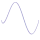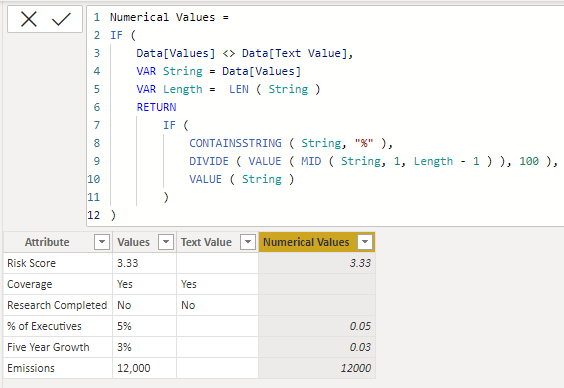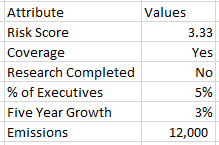cancel
Showing results for
Did you mean:Helper I

## Extracting text and numerical types from one column and splitting into two columns

I have an interesting problem I am hoping the community can solve.

The first part of the problem is how do I split one column into two columns dependant on the data type i.e.:

I have this flattened view:

 Attribute Values Risk Score 3.33 Coverage Yes Research Completed No % of Executives 5% Five Year Growth 3% Emissions 12,000

I would prefer the below unless someone can solve the second potential issue.

 Attribute Values Text Values Numerical Values Risk Score 3.33 3.33 Coverage Yes Yes Research Completed No No % of Executives 5% 5% Five Year Growth 3% 3% Emissions 12,000 12,000

My second potential issue is that if I am left with only the value column, how do I get the SUM function to work.

When there are mixed type of data it does not work, is there any work around so it only counts the numerical rows?

Best Regards,

1 ACCEPTED SOLUTIONHelper I

After trying all the methods the most workable solution was as follows:

1. Duplicate the column you want to split

2. Change the duplicated column to a numerical type column

3. Replace any errors with nulls

4. Create a conditional column that states where the numerical type column is 'null' then take the value from the original column.

7 REPLIES 7Helper I

After trying all the methods the most workable solution was as follows:

1. Duplicate the column you want to split

2. Change the duplicated column to a numerical type column

3. Replace any errors with nulls

4. Create a conditional column that states where the numerical type column is 'null' then take the value from the original column.Super User

Just FYI, this is nearly the same as what I suggested except that I did the equivalent of 1,2,3 as single step, #"Added Numerical Column".Community Support

Is your problem being solved. If so, please mark it as a solution so that more people can see it.

Best Regards

Community Support Team _ chenwu zhuSuper User

This can be done pretty simply in the Query Editor using the Number.FromText() function. Anything that throws an error when trying to convert to a number we can consider as text. To get the numerical column, this should be sufficient as a new custom column:

``try Number.FromText([Values]) otherwise null``

Sample query you can paste into the Advanced Editor of a new blank query:

``````let
Source = Table.FromRows(Json.Document(Binary.Decompress(Binary.FromText("JY3BCsIwEER/ZVnoLUg0+AWlevNQTyXkUOJqg9aVbNr6+SbxOPPeMNZiH+QJV8+RUKHZGYNOWWx5pTg+SjeQ1KonoTH6CVqePy9KdMvwwpU1wHfovuSXFNbsKzw2FZxyhCHv4Bx5S1P5+JNuDiKB30XeH5TWGp37AQ==", BinaryEncoding.Base64), Compression.Deflate)), let _t = ((type nullable text) meta [Serialized.Text = true]) in type table [Attribute = _t, Values = _t]),
#"Added Numerical Column" = Table.AddColumn(Source, "Numerical", each try Number.FromText([Values]) otherwise null, type number),
#"Added Text Column" = Table.AddColumn(#"Added Numerical Column", "Text", each if [Numerical] = null then [Values] else null, type text)
inSuper User

Hi @knowledgegarage
You can use dax to create two calculated columns as follow https://www.dropbox.com/t/qOO0oQXdjDWteLOH``````Text Value =
VAR T1 = UNION ( GENERATESERIES ( 0, 9, 1 ), { ".", "%", "," } )
VAR String = Data[Values]
VAR Length = LEN ( String )
VAR T2 = GENERATESERIES ( 1, Length, 1 )
VAR T3 = ADDCOLUMNS ( T2, "@Letter", MID ( String, [Value], 1 ) )
VAR T4 = FILTER ( T3, NOT ( [@Letter] IN T1 ) )
RETURN
CONCATENATEX ( T4, [@Letter] )``````
``````Numerical Values =
IF (
Data[Values] <> Data[Text Value],
VAR String = Data[Values]
VAR Length =  LEN ( String )
RETURN
IF (
CONTAINSSTRING ( String, "%" ),
DIVIDE ( VALUE ( MID ( String, 1, Length - 1 ) ), 100 ),
VALUE ( String )
)
)``````Helper I

No. It was like so:I want to either split it into two columns like so unless there is another way to calculate.Super User

What was the original shape of the column? Something like "Risk Score 3.33" or additionaly there are brackets/special characters? Would you mind a DAX solution?